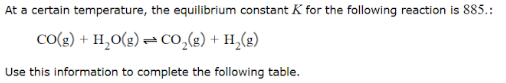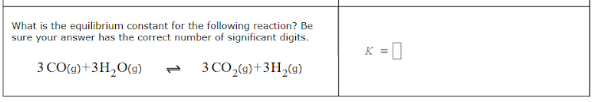# At a certain temperature, the equilibrium constant K for the following reaction is 885.: CO(g) + H20(g) ⇌ CO2(g) + H2(g) Use this information to complete the following table. What is the equilibrium constant for the following reaction? Be sure your answer has the correct number of significant digits. 3 CO(g) + 3HO(g) ⇌ 3 CO2(g) + 3H2(g)